# Portfolio Analysis: Calculating Risk and Returns, Strategies and More

### Introduction

The process of trading is a complex one with a number of steps like stocks selection, the formation of strategies, and creation of a portfolio and so on. Here, we will focus on one such step which is computing the expected returns and variances for a portfolio having n number of stocks.

#### Expected return on a single stock

The expected return of a portfolio provides an estimate of how much return one can get from their portfolio. And variance gives the estimate of the risk that an investor is taking while holding that portfolio. The returns and the risk of the portfolio depending on the returns and risks of the individual stocks and their corresponding shares in the portfolio.

The parameters of the risk and return of any stock explicitly belong to that particular stock, however, the investor can adjust the return to risk ratio of his/ her portfolio to the desired level using certain measures. One such measure is to adjust the weights of the stocks in the investors’ portfolio.

Here we will discuss how the weights of individual stocks impact these two parameters of the portfolio. Consider a stock ABC. Let ri be the expected return on the stock and rx be any return having a probability of px. The expected return, ri, can be computed using the below equation.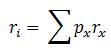Let us assume that ABC can generate the returns as per column A with corresponding probabilities given in column B. The expected return from ABC can be computed as shown in column C, which is the product of columns A and B.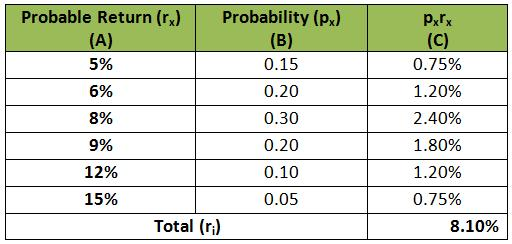Let us look at the code to compute the expected return of this stock. We start by importing the pandas library.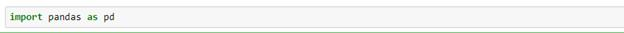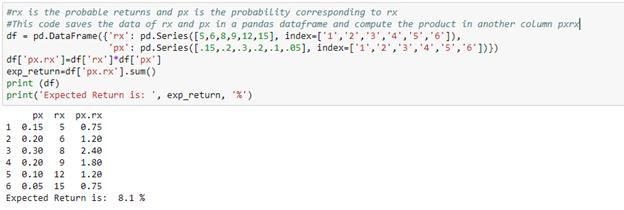The expected return on the stock is 8.10% as per the calculations shown above. The returns in column A can be computed using Capital Asset Pricing Model (CAPM).

#### Risk (or variance) on a single stock

The variance of the return on stock ABC can be calculated using the below equation.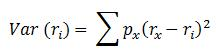The following table gives the computation of the variance using the same example above.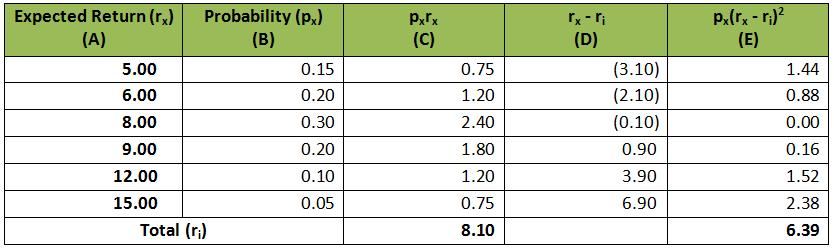We can compute the variance of the single stock using python as: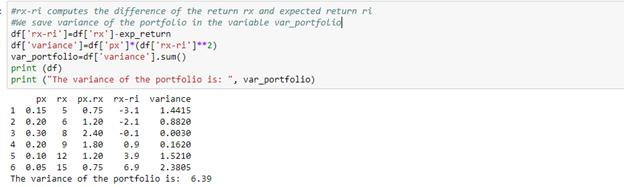Hence, the variance of return of the ABC is 6.39. The standard deviation of the returns can be calculated as the square root of the variance.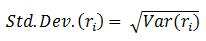Having computed the expected return and variance for the stock, we will now see how to calculate the return and variance of the portfolio. We use the expected return and variance of the portfolio to optimize it. We can adjust the weights of the stocks to maximize the return and minimize the standard deviation.

#### Expected return on an n-stock portfolio

Let us take an n-stock portfolio. Assume that the expected return from ith stock is ri. The expected return on the portfolio will then be: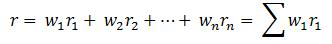The weight of any stock is the ratio of the amount invested in that stock to the total amount invested. For the below portfolio, the weights are shown in the table.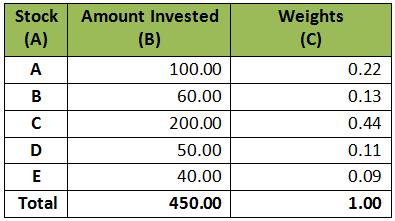Let us see how we can compute the weights using python for this portfolio.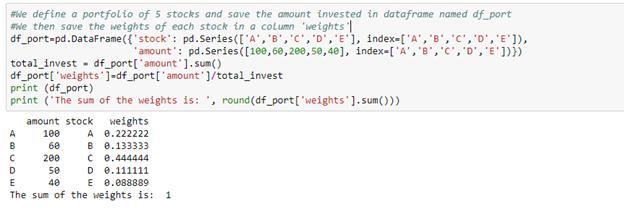The sum of the weights of all the stocks in the portfolio will always be 1. Next, we will see the expected return on this portfolio.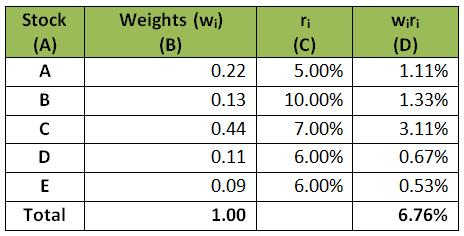The expected return of the portfolio can be computed as: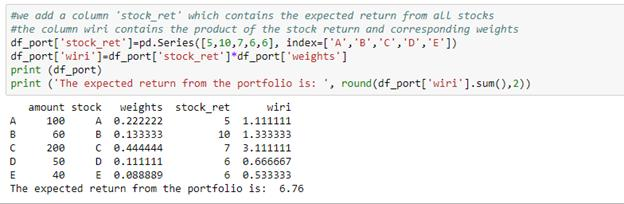#### Covariances and correlation of returns

Before moving on to the variance of the portfolio, we will have a quick look at the definitions of covariance and correlation. Covariance (or correlation) denotes the directional relationship of the returns from any two stocks. The magnitude of the covariance denotes the strength of the relationship. If covariance (or correlation) is zero, there is no relation and if the sign of covariance (or correlation) is negative, it indicates that if one stock moves in one direction the other will move in the other direction. The equations to compute the covariance and correlation are given below.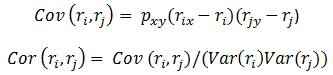#### Box method for Variance of a portfolio

For variance of the portfolio, we will use box method. For an n-stock portfolio, we will create an n X n matrix with all the stocks on X and Y axes as illustrated below. Each cell contains the product of the weights of the corresponding column and the covariance of the corresponding stocks.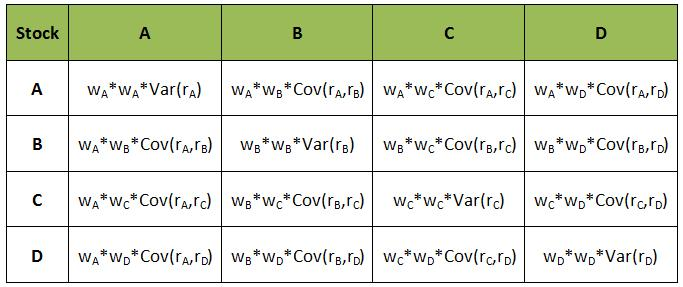Let us look at the stepwise procedure to use the box method for variance computation.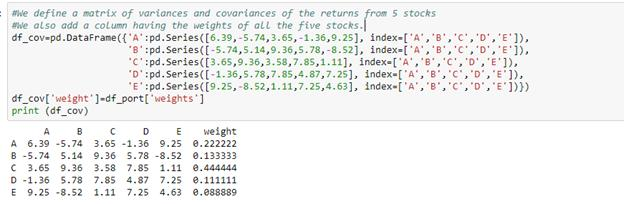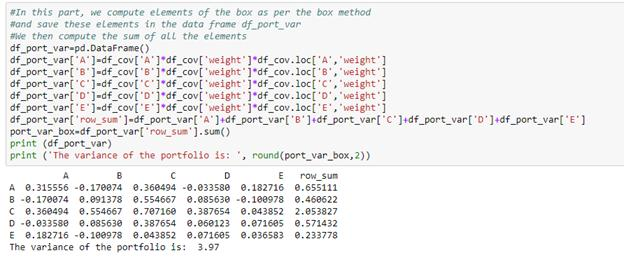The variance of the portfolio will be the sum of all the cells of this table. Hence, the variance of a two stock portfolio will be: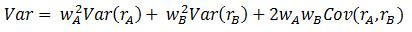#### Special case of fully diversified portfolio

Now we will take a special case in which there are n stocks and the weights of all the stocks are equal. Hence, the weight of each stock will be 1/n. Using the above box method, we can write the sum of all the diagonal elements as: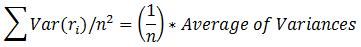After removing n diagonal elements, we are left with n2 – n elements. The sum of the remaining elements can be written as: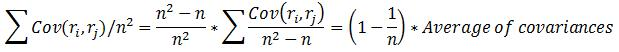Hence, the variance of such a portfolio will be: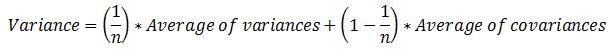For a fully diversified portfolio, we can assume that we have added every possible stock in our portfolio. Therefore, n will tend to infinity and 1/n will tend to zero. So, the variance of a fully diversified portfolio will be the average of the covariances. So, we can say that diversification eliminates all risks except the covariances of the stocks, which is the market risk.

### What Next?

If you want to learn various aspects of Algorithmic trading then check out the Executive Programme in Algorithmic Trading (EPAT™). The course covers training modules like Statistics & Econometrics, Financial Computing & Technology, and Algorithmic & Quantitative Trading. EPAT™ equips you with the required skill sets to be a successful trader. Enroll now!